# ISBLANK Function

Checks if a specified cell is blank or not

## What is Excel ISBLANK Function?

The ISBLANK function is an Excel Information function, which returns true if the argument cell has no information in it. ISBLANK checks a specified cell and tells us if it is blank or not. If it is blank, it will return TRUE; else, it will return FALSE. The function was introduced in MS Excel 2007.

In financial analysis, we deal with data all the time. The ISBLANK function is useful in checking if a cell is blank or not. For example, if A5 contains a formula that returns an empty string “” as a result, the function will return FALSE. Thus, it helps in removing both regular and non-breaking space characters.

However, if a cell contains good data, as well as non-breaking spaces, it is possible to remove the non-breaking spaces from the data.

### Formula

=ISBLANK(value)

Where:

• Value (required argument) is the value that we wish to test. (This function takes in a cell)

### How to use the Excel ISBLANK Function

As a worksheet function, ISBLANK can be entered as part of a formula in a cell of a worksheet. To understand the uses of this function, let us consider a few examples:

#### Highlight Missing Values – Example

Suppose we are given the following data: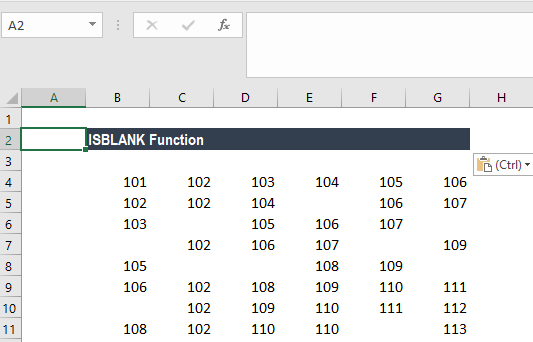Suppose we wish to highlight cells which are empty, we can use the ISBLANK coupled with conditional formatting. For example, suppose we want to highlight the blank cells in the range A2:F9, we select the range and use a conditional formatting rule with the following formula: =ISBLANK(A2:F9).

##### How to do conditional formatting?

Under Home tab – Styles, click on Conditional Formatting. Then click on New Rule and then select – Use a Formula to determine which cells to format: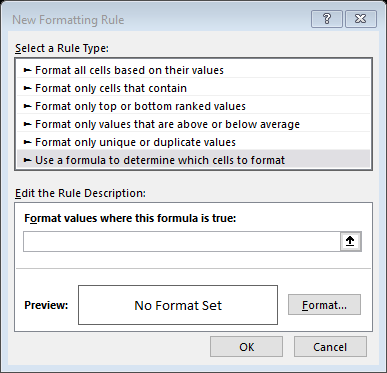The input formula is shown below: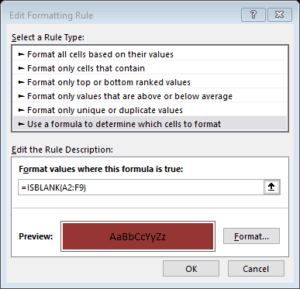We will get the results below.

Conditional formatting didn’t highlight cell E5. After checking, there is a formula inserted into the cell.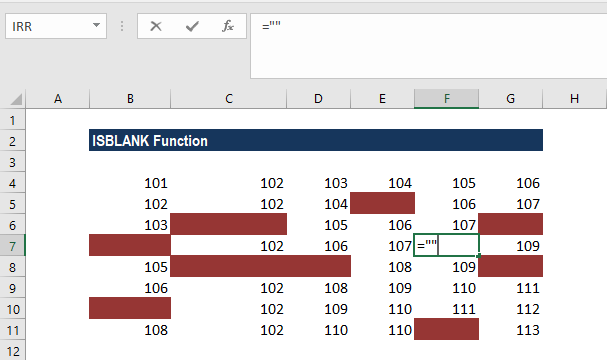The Excel ISBLANK function will return TRUE when a cell is actually empty. If a cell is an empty string (“”), ISBLANK will return FALSE, as it is not technically blank,  and it won’t be highlighted as shown above.

#### Extracting the first NON-Blank value in an array

Suppose we wish to get the first non-blank value (text or number) in a one-row range, we can use an array formula based on the INDEX, MATCH, and ISBLANK functions.

We are given the data below: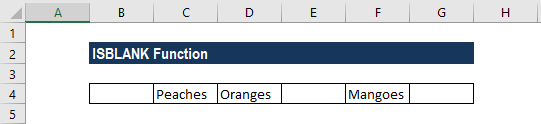Here, we want to get the first non-blank cell, but we don’t have a direct way to do that in Excel. We could use VLOOKUP with a wildcard *, but that will only work for text, not numbers.

Hence, we need to build the functionality by nesting formulas. One way to do it is to use an array function that “tests” cells and returns an array of TRUE/FALSE values that we can then match with MATCH. Now MATCH looks for FALSE inside the array and returns the position of the first match found, which, in this case, is 2. Now the INDEX function takes over and gets the value at position 2 in the array, which, in this case, is the value PEACHES.

As this is an array formula, we need to enter it with CTRL+SHIFT+ENTER.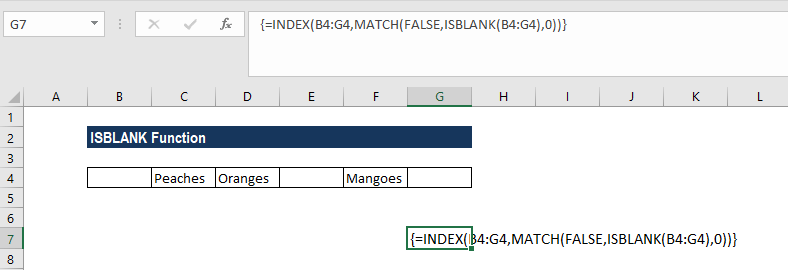We get the results below: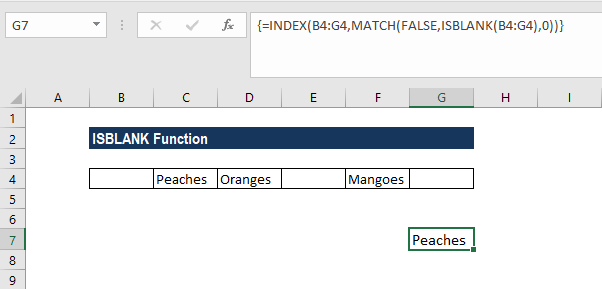Thanks for reading CFI’s guide to important Excel functions! By taking the time to learn and master these functions, you’ll significantly speed up your financial modeling and valuation analysis. To learn more, check out these additional CFI resources:

• Excel Functions for Finance